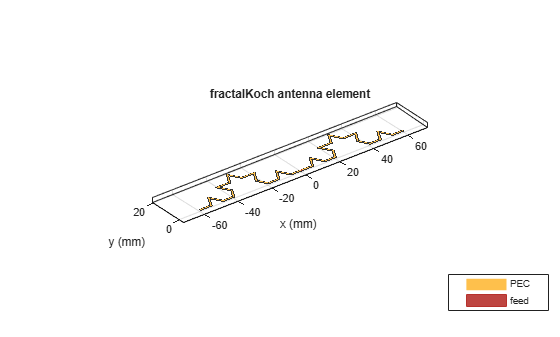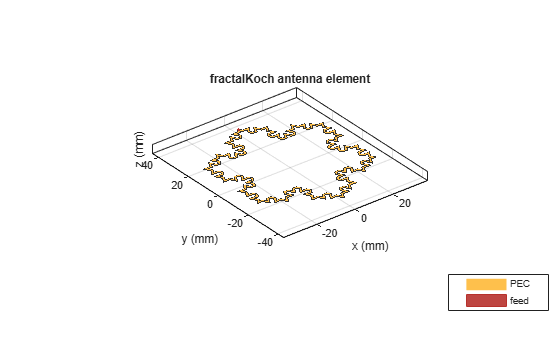fractalKoch

Create Koch curve fractal dipole or loop antenna on xy- plane

Description

The fractalKoch object creates a Koch curve fractal dipole or loop antenna on an xy- plane. These fractals are used in multiband and wideband applications like Global System for Mobile Communications (GSM), Universal Mobile Telecommunication Service (UMTS), and Bluetooth.A fractal antenna uses a self-similar design to maximize the length or increase the perimeter of a material that transmits or receives electromagnetic radiation within a given volume or area. The main advantage of fractal antennas is that they are compact, which is an important requirement for small and complex circuits. Fractal antennas also have more input impedance or resistance due to increased length or perimeter, respectively.

All fractal antennas are printed structures that are etched on a dielectric substrate.

Creation

Description

example

ant = fractalKoch creates a Koch curve fractal antenna on an X-Y plane. The default is a dipole with Koch curve length chosen for an operating frequency of 0.86 GHz.

example

ant = fractalKoch(Name,Value) sets properties using one or more name-value pairs. For example, ant = fractalKoch('NumIterations',4) creates a Koch curve fractal antenna with four iterations. Enclose each property name in quotes.

Properties

expand all

Number of iterations of the fractal antenna, specified as a scalar integer.

Example: 'NumIterations',2

Example: ant.NumIterations = 2

Data Types: double

Length of the Koch curve along the x-axis, specified as a positive scalar integer in meters.

Example: 'Length',0.5000

Example: ant.Length = 0.5000

Data Types: double

Width of the Koch curve along y-axis, specified as a positive scalar integer in meters.

Example: 'Width',0.0050

Example: ant.Width = 0.0050

Data Types: double

Type of Koch configuration, specified as 'dipole' or 'loop'.

Example: 'Type','loop'

Example: ant.Type = 'loop'

Data Types: char | string

Type of the metal used as a conductor, specified as a metal material object. You can choose any metal from the MetalCatalog or specify a metal of your choice. For more information, see metal. For more information on metal conductor meshing, see Meshing.

Example: m = metal('Copper'); 'Conductor',m

Example: m = metal('Copper'); ant.Conductor = m

Lumped elements added to the antenna feed, specified as a lumped element object. You can add a load anywhere on the surface of the antenna. By default, the load is at the origin. For more information, see lumpedElement.

Example: 'Load',lumpedelement. lumpedelement is the object for the load created using lumpedElement.

Tilt angle of the antenna, specified as a scalar or vector with each element unit in degrees. For more information, see Rotate Antennas and Arrays.

Example: 'Tilt',90

Example: ant.Tilt = 90

Example: 'Tilt',[90 90],'TiltAxis',[0 1 0;0 1 1] tilts the antenna at 90 degrees about the two axes defined by the vectors.

Note

The wireStack antenna object only accepts the dot method to change its properties.

Data Types: double

Tilt axis of the antenna, specified as:

• Three-element vector of Cartesian coordinates in meters. In this case, each coordinate in the vector starts at the origin and lies along the specified points on the X-, Y-, and Z-axes.

• Two points in space, each specified as three-element vectors of Cartesian coordinates. In this case, the antenna rotates around the line joining the two points in space.

• A string input describing simple rotations around one of the principal axes, 'X', 'Y', or 'Z'.

Example: 'TiltAxis',[0 1 0]

Example: 'TiltAxis',[0 0 0;0 1 0]

Example: ant.TiltAxis = 'Z'

Note

The wireStack antenna object only accepts the dot method to change its properties.

Data Types: double

Object Functions

 show Display antenna or array structure; display shape as filled patch axialRatio Axial ratio of antenna beamwidth Beamwidth of antenna charge Charge distribution on metal or dielectric antenna or array surface current Current distribution on metal or dielectric antenna or array surface design Design prototype antenna or arrays for resonance around specified frequency efficiency Radiation efficiency of antenna EHfields Electric and magnetic fields of antennas; Embedded electric and magnetic fields of antenna element in arrays impedance Input impedance of antenna; scan impedance of array mesh Mesh properties of metal or dielectric antenna or array structure meshconfig Change mesh mode of antenna structure optimize Optimize antenna or array using SADEA optimizer pattern Radiation pattern and phase of antenna or array; Embedded pattern of antenna element in array patternAzimuth Azimuth pattern of antenna or array patternElevation Elevation pattern of antenna or array rcs Calculate and plot radar cross section (RCS) of platform, antenna, or array returnLoss Return loss of antenna; scan return loss of array sparameters Calculate S-parameter for antenna and antenna array objects vswr Voltage standing wave ratio of antenna

Examples

collapse all

Create and view a default Koch curve fractal antenna.

ant = fractalKoch
ant =
fractalKoch with properties:

NumIterations: 2
Length: 0.0600
Width: 1.0000e-03
Type: 'dipole'
Conductor: [1x1 metal]
Tilt: 0
TiltAxis: [1 0 0]

show(ant)Create and view a Koch loop fractal antenna with three iterations.

ant = fractalKoch('NumIterations',3,'Type','loop');
show(ant)